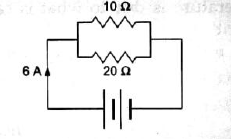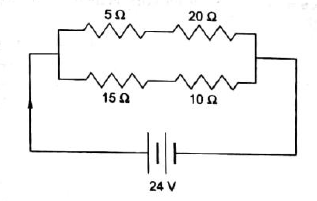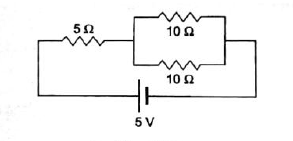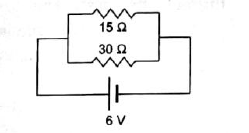# Electricity Class10 CBSE - MCQ test 3

Electricity Class10 CBSE - MCQ test 3
Q1. Two rasister of 10Ω & 20Ω are connected in parallel in in circuit as given below. What is value of current in 20Ω rasistence?4A

2A

3A

5A

Q2. What is the value of total rasistence of circuit shown below.12.5 Ω

50 Ω

25 Ω

None of these

Q3. What is be current in circuit by 5V cell shown below .1A

0.5A

2A

None

Q4. What is the equvalent rasistance of circuit shown below.5 Ω

10 Ω

45 Ω

None of these

Q5.The equivalent rasistence of two rasistence connected in parallel is ..... than each rasistance.

Less

More

Half

All of the above

Q6. Three raistence 2Ω are connected in series in a circuit with a battery and current in each rasistance is 2A. What is value of potential diiference of battery.

6V

12V

10V

None of these

Q7. A thick wre has more rasistence than a thin wire of same material.

True

False

Q8.Two equal rasistence are connected in parallel ,the equivalent rasistence is havlved.

True

False

Q9. An electron moves from A to B.The potential of point A is higher than point B.

True

False

Q10.An charge moves from A to B and workdone per unit charge is called .

poetential of point A

poetential of pointB

poetential difference of point B to point A

None of these

Q11. There are two statements given below:
A. In series combination of rasistors ,same current flows throgh all rasisters.
B.In parallel combination of rasistors , same potential diffrence exists accors all rasisters.

Both (A) and (B) are incorrect.

Both (A) and (B) are correct.

(A) corect and (B) incorrect.

same in all the cases

Q12. Current in a wire depend upon

Potential diffrence accross the wire

Rasistance of wire

Both

None of these

Q13. Free electrons of a metal are free to ......

move on surface.

escape thrugh the surface.

free to fall into nuclei.

move inside the metal.

Q14.An electrical appliance has a resistance of 25 Ω. When this electrical appliance is connected to a 230 V supply line, the current passing through it will be:

92A

9.2A

2.9A

29A

Q15. Keeping the potential difference constant, the resistance of the circuit is halved. The current will become:

One-fourth

One-fourth

Double

None of these

Q16.When a 4 Ω resistor is connected across the terminals of a 2 V battery, the number of coulombs passing through the resistor per second is:

0.5

1

2

3

Q17. A wire of resistance R1 is cut into five equal pieces. These five pieces of wire are then connected in parallel. If the resultant resistance of this combination be R2, then the ratio R1/ R2 is:

1/25

1/5

5

25

Q18. The current flow in conductor is due to:

free electrons

protons

ions

neutorn

Q19. Ohm’s law is true for:

metallic conductor

non-metallic conductor

semi-conductor

All of these

Q20. Charge on one electron is:

–9.1 × 10^-19 C

–1.6 × 10^-19 C

1.6 × 10^-19 C

none of these